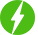# Data Science and Machine Learning Basic to Advanced

Deal Score0
Free \$69.99
Deal Score0
Free \$69.99

Data Science and Machine Learning Basic to Advanced, Complete Introduction to Data Science and Machine Learning from Basic to Advanced.

• Learn how to use Numpy and Pandas for Data Analysis. This will cover all basic concepts of Numpy and Pandas that are useful in data analysis.
• Learn to create impactful visualizations using Matplotlib and Seaborn. Creating impactful visualizations is a crucial step in developing a better understanding about your data.
• This course covers all Data Preprocessing steps like working with missing values, Feature Encoding and Feature Scaling.
• Learn about different Machine Learning Models like Random Forest, Decision Trees, KNN, SVM, Linear Regression, Logistic regression etc… All the video sessions will first discuss the basic theory concept behind these algorithms followed by the practical implementation.
• Learn to how to choose the best hyper parameters for your Machine Learning Model using GridSearch CV. Choosing the best hyper parameters is an important step in increasing the accuracy of your Machine Learning Model.
• You will learn to build a complete Machine Learning Pipeline from Data collection to Data Preprocessing to Model Building. ML Pipeline is an important concept that is extensively used while building large scale ML projects.
• This course has two projects at the end that will be built using all concepts taught in this course. The first project is about Diabetes Prediction using a classification machine learning algorithm and second is about prediciting the insurance premium using a regression machine learning algorithm.
We will be happy to hear your thoughts• Total (0)
0